GeeksforGeeks App
Open AppBrowser
Continue

# Minimum difference between the highest and the smallest value of mines distributed

Given n companies and m oil mines having values, the task is to distribute the mines among n companies in a fair manner. That is the difference between the company getting the highest sum of values of mines and the one getting the lowest should be minimum. Compute the minimum difference. Note that oil mines distributed to each company should be adjacent to each other. Also,Examples:

Input: n = 2, m = 4 values of mines = [6, 10, 13, 2]
Output: 1 –> mines distributed as {(6, 10), (13, 2)}, hence output is (6+10) – (13+2) = 1

Input: n = 3, m = 4 values of mines = [6, 10, 13, 2]
Output: 9 –> mines distributed as {(6), (10), (13, 2)}, hence output is (13+2) – (6) = 9

Source: Samsung RnD 3 hrs coding round question

## Recommended: Please try your approach on {IDE} first, before moving on to the solution.

Approach:

1. Construct an array such that value at each index contains the sum of current and all previous values in the array.
2. Include the last value in the array in our solution array.
3. Recursively construct all the possible solution arrays by selecting n-1 values from the given m-1 values starting from the second last index (As the last value in the array has already been included in our solution array).
4. Subtract the value at next index from the value at current index in the solution array for values at each index except the last value. Calculate the difference between the highest and the lowest value for all the solution arrays and return the minimum.

Let’s take an example to understand the above approach step by step: Initially, the input is provided as below: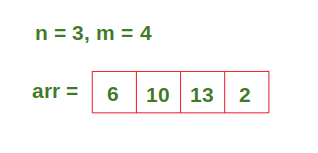After Step 1, our array looks like the array given below :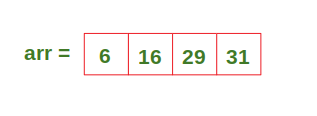After Step 2, the solution array looks like the array given below: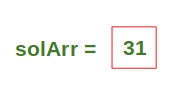After Step 3, we get the following possible solution arrays: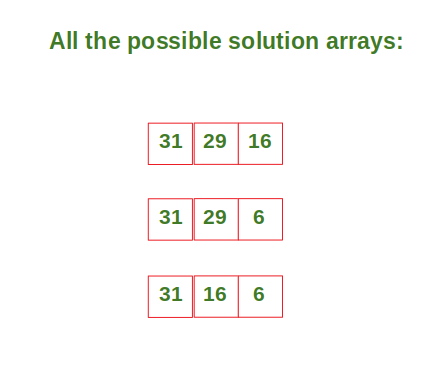After Step 4, our solution arrays look like the arrays given below. Then we compute the difference between the maximum and minimum value in the array for all the arrays and return the one which is minimum (in this case 9).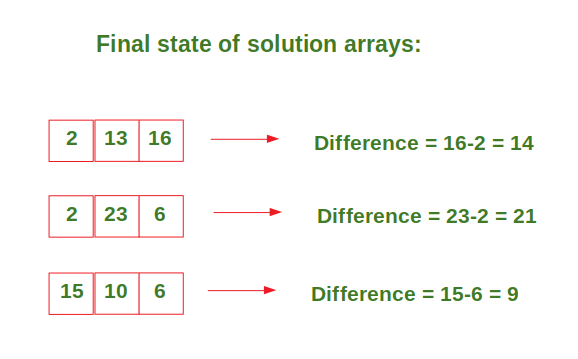Below is the implementation of the above approach:

## C++

 `#include ``#include ``#include ` `using` `namespace` `std;` `// This function constructs the solution array``// recursively and returns the difference``// between the highest and lowest value.``int` `Func(vector<``int``> solArr, vector<``int``> arr, ``int` `index, ``int` `n)``{``    ``// If the values have been distributed,``    ``// compute the difference``    ``if` `(n == 0)``    ``{``        ``// Compute the difference between the``        ``// highest and lowest values in the solution array``        ``for` `(``int` `i = 0; i < solArr.size() - 1; i++)``        ``{``            ``solArr[i] = solArr[i] - solArr[i + 1];``        ``}``        ``return` `*max_element(solArr.begin(), solArr.end()) - *min_element(solArr.begin(), solArr.end());``    ``}``    ``else``    ``{``        ``// If the solution array hasn't been fully``        ``// constructed, we need to continue building it` `        ``// solArr can be constructed even if we``        ``// don't include the current value``        ``if` `(index >= n)``        ``{``            ``// Two recursive calls: one with the current``            ``// value included in the solution array, and``            ``// one without it included``            ``solArr.push_back(arr[index]);``            ``int` `diff1 = Func(solArr, arr, index - 1, n - 1);``            ``solArr.pop_back();``            ``int` `diff2 = Func(solArr, arr, index - 1, n);``            ``return` `min(diff1, diff2);``        ``}``        ``else``        ``{``            ``// solArr can't be constructed hence``            ``// we have to include the current value``            ``solArr.push_back(arr[index]);``            ``return` `Func(solArr, arr, index - 1, n - 1);``        ``}``    ``}``}` `int` `main()``{``    ``int` `n = 3;``    ``vector<``int``> arr = { 6, 10, 13, 2 };` `    ``// Construct array such that value at each index``    ``// contains the sum of current and all previous``    ``// values in the array.``    ``for` `(``int` `i = 1; i < arr.size(); i++)``    ``{``        ``arr[i] += arr[i - 1];``    ``}` `    ``// Include the last value of the array in our solution array.``    ``vector<``int``> solArr = { arr[arr.size() - 1] };` `    ``// Call the Func function to compute the difference``    ``// between the highest and lowest values in the solution array``    ``cout << Func(solArr, arr, arr.size() - 2, n - 1) << endl;` `    ``return` `0;``}``// This code is contributed by divyansh2212`

## Java

 `import` `java.util.*;` `public` `class` `Main {` `  ``// This function constructs the solution array``  ``// recursively and returns the difference``  ``// between the highest and lowest value.``  ``static` `int` `func(List solArr, ``int``[] arr,``                  ``int` `index, ``int` `n)``  ``{` `    ``// If the values have been distributed,``    ``// compute the difference``    ``if` `(n == ``0``)``    ``{` `      ``// Compute the difference between the``      ``// highest and lowest values in the solution array``      ``for` `(``int` `i = ``0``; i < solArr.size() - ``1``; i++) {``        ``solArr.set(i, solArr.get(i) - solArr.get(i + ``1``));``      ``}``      ``return` `Collections.max(solArr) - Collections.min(solArr);``    ``}``    ``else``    ``{` `      ``// solArr can be constructed even if we``      ``// don't include the current value``      ``if` `(index >= n)``      ``{` `        ``// Two recursive calls: one with the current``        ``// value included in the solution array, and``        ``// one without it included``        ``return` `Math.min(``          ``func(``new` `ArrayList(solArr) {{add(arr[index]);}}, arr, index - ``1``, n - ``1``),``          ``func(``new` `ArrayList(solArr), arr, index - ``1``, n)``        ``);``      ``}``      ``else``      ``{` `        ``// solArr can't be constructed hence``        ``// we have to include the current value``        ``return` `func(``new` `ArrayList(solArr) {{add(arr[index]);}}, arr, index - ``1``, n - ``1``);``      ``}``    ``}``  ``}` `  ``public` `static` `void` `main(String[] args) {``    ``int` `n = ``3``;``    ``int``[] arr = {``6``, ``10``, ``13``, ``2``};` `    ``// Construct array such that value at each index``    ``// contains the sum of current and all previous``    ``// values in the array.``    ``for` `(``int` `i = ``1``; i < arr.length; i++) {``      ``arr[i] += arr[i - ``1``];``    ``}` `    ``// Include the last value of the array in our solution array.``    ``List solArr = ``new` `ArrayList() {{ add(arr[arr.length - ``1``]); }};` `    ``// Call the func function to compute the difference``    ``// between the highest and lowest values in the solution array``    ``System.out.println(func(``new` `ArrayList(solArr), arr, arr.length - ``2``, n - ``1``));``  ``}``}`

## Python3

 `# Python3 code to minimize the difference``# between the highest and lowest value containing company` `# This function constructs the solution array``# recursively and returns the difference``# between the highest and lowest value.``def` `func(solArr, arr, index, n):``    ` `    ``# If the values have been distributed,``    ``# compute the difference``    ``if` `n ``=``=` `0``:``        ``for` `i ``in` `range``(``len``(solArr)``-``1``):``            ``solArr[i] ``=` `solArr[i] ``-` `solArr[i ``+` `1``]``            ` `        ``return` `max``(solArr) ``-` `min``(solArr)``        ` `    ``else``:``        ` `        ``# solArr can be constructed even if we``        ``# don't include the current value``        ``if` `index >``=` `n:``            ``return` `min``(func(solArr[:] ``+` `[arr[index]], arr, index``-``1``, n``-``1``),``                    ``func(solArr[:], arr, index``-``1``, n))``        ` `        ``# solArr can't be constructed hence``        ``# we have to include the current value   ``        ``else``:``            ``return` `func(solArr[:] ``+` `[arr[index]], arr, index``-``1``, n``-``1``)``            ` `n ``=` `3``arr ``=` `[``6``, ``10``, ``13``, ``2``]` `# Construct array such that value at each index``# contains the sum of current and all previous``# values in the array.``for` `i ``in` `range``(``1``, ``len``(arr)):``    ``arr[i] ``+``=` `arr[i``-``1``]` `# Include the last value of``# the array in our solution array.``solArr ``=` `[arr[``-``1``]]``    ` `print``(func(solArr[:], arr, ``len``(arr)``-``2``, n``-``1``))`

## C#

 `using` `System;``using` `System.Linq;` `class` `Program``{``  ``// This function constructs the solution array``  ``// recursively and returns the difference``  ``// between the highest and lowest value.``  ``static` `int` `Func(``int``[] solArr, ``int``[] arr, ``int` `index, ``int` `n)``  ``{``    ` `    ``// If the values have been distributed,``    ``// compute the difference``    ``if` `(n == 0)``    ``{``      ` `      ``// Compute the difference between the``      ``// highest and lowest values in the solution array``      ``for` `(``int` `i = 0; i < solArr.Length - 1; i++)``      ``{``        ``solArr[i] = solArr[i] - solArr[i + 1];``      ``}``      ``return` `solArr.Max() - solArr.Min();``    ``}``    ``else``    ``{``      ``// If the solution array hasn't been fully``      ``// constructed, we need to continue building it` `      ``// solArr can be constructed even if we``      ``// don't include the current value``      ``if` `(index >= n)``      ``{``        ``// Two recursive calls: one with the current``        ``// value included in the solution array, and``        ``// one without it included``        ``return` `Math.Min(``          ``Func(solArr.Concat(``new``[] { arr[index] }).ToArray(), arr, index - 1, n - 1),``          ``Func(solArr.ToArray(), arr, index - 1, n)``        ``);``      ``}``      ``else``      ``{``        ``// solArr can't be constructed hence``        ``// we have to include the current value``        ``return` `Func(solArr.Concat(``new``[] { arr[index] }).ToArray(), arr, index - 1, n - 1);``      ``}``    ``}``  ``}` `  ``static` `void` `Main(``string``[] args)``  ``{``    ``int` `n = 3;``    ``int``[] arr = { 6, 10, 13, 2 };` `    ``// Construct array such that value at each index``    ``// contains the sum of current and all previous``    ``// values in the array.``    ``for` `(``int` `i = 1; i < arr.Length; i++)``    ``{``      ``arr[i] += arr[i - 1];``    ``}` `    ``// Include the last value of the array in our solution array.``    ``int``[] solArr = { arr[arr.Length - 1] };` `    ``// Call the Func function to compute the difference``    ``// between the highest and lowest values in the solution array``    ``Console.WriteLine(Func(solArr.ToArray(), arr, arr.Length - 2, n - 1));``  ``}``}`

## Javascript

 `// JavaScript code to minimize the difference``// between the highest and lowest value containing company` `// This function constructs the solution array``// recursively and returns the difference``// between the highest and lowest value.``function` `func(solArr, arr, index, n)``{` `// If the values have been distributed,``// compute the difference``if` `(n == 0) {``for` `(let i = 0; i < solArr.length - 1; i++) {``solArr[i] = solArr[i] - solArr[i + 1];``}``return` `Math.max(...solArr) - Math.min(...solArr);``} ``else` `{` `// solArr can be constructed even if we``// don't include the current value``if` `(index >= n) {``return` `Math.min(``func(solArr.concat(arr[index]), arr, index - 1, n - 1),``func(solArr.slice(), arr, index - 1, n)``);``} ``else` `{` `// solArr can't be constructed hence``// we have to include the current value``return` `func(solArr.concat(arr[index]), arr, index - 1, n - 1);``}``}``}` `const n = 3;``const arr = [6, 10, 13, 2];` `// Construct array such that value at each index``// contains the sum of current and all previous``// values in the array.``for` `(let i = 1; i < arr.length; i++) {``arr[i] += arr[i - 1];``}` `// Include the last value of``// the array in our solution array.``const solArr = [arr[arr.length - 1]];` `console.log(func(solArr.slice(), arr, arr.length - 2, n - 1));`

Output

`9`
• Time Complexity:• Space Complexity:My Personal Notes arrow_drop_up